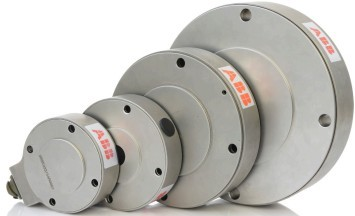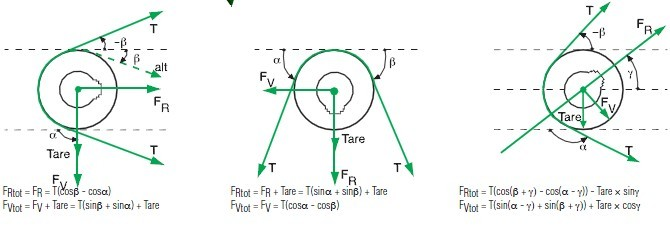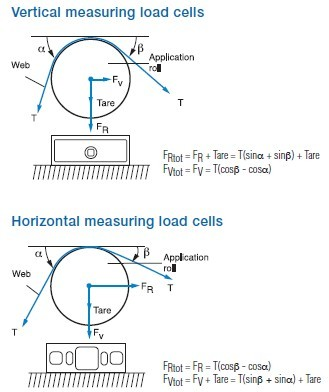﻿ ABB纸幅张力测定概述-深圳市励德恒业科技有限公司－官网

### ABB纸幅张力测定概述

• 品牌：   ABB
• 型号：   PFTL101A

ABB纸幅张力测定系统概述

，大多数机械应用领域都意识到了卷纸机张力测量是设备成功运行的基本要求，使张力测量的好与坏直接体现在产品加工的质量和运行效率的结果之上。

ABB纸幅张力测定系统基于Pressductor技术，极大的改变了这一状况，为客户提供高质量的张力测量产品，使各种行业的客户体会到系统优点带来的益处，在造纸和金属加工行业畅销40多年。

ABB纸幅张力测定系统主要包括：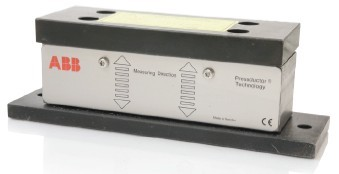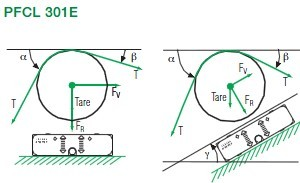FRtot =FR+Tare                   FRtot =FR+Tare×cosγ
=T(sinα+sinβ)+Tare              =T(sin(α-γ)+sin(β+γ))+Tare×cosγ
FVtot =FV                           FVtot =FV-Tare×sinγ
=T(cosβ-cosα)                     =T(cos(β+γ)-cos(α-γ))-Tare×sinγ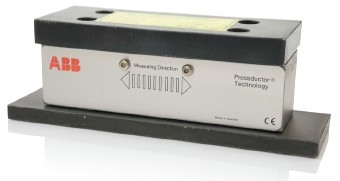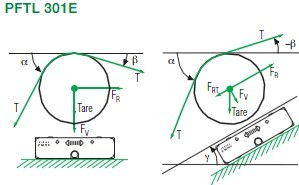FRtot =FR+Tare                  FRtot =FR+Tare×cosγ
=T(sinα+sinβ)+Tare             =T(sin(α-γ)+sin(β+γ))+Tare×cosγ
FVtot =FV                          FVtot =FV-Tare×sinγ
=T(cosβ-cosα)                    =T(cos(β+γ)-cos(α-γ))-Tare×sinγ